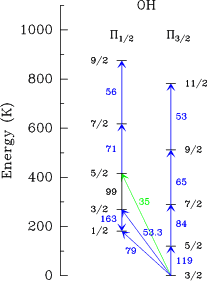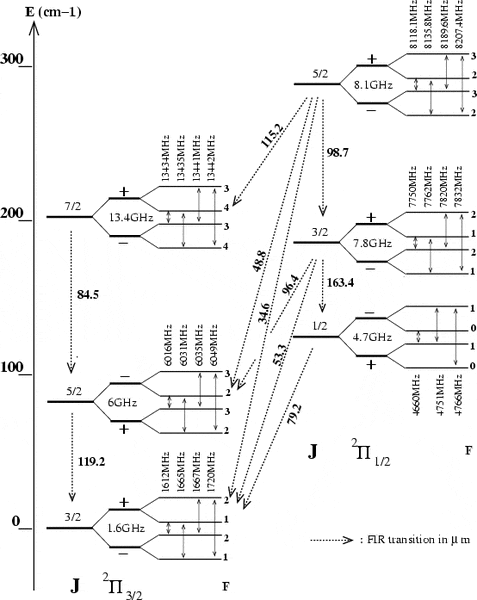# Determine the Transition Wavelength

Hi all, the question is as follows:

1. Homework Statement

From the energy level diagram for OH in the study guide, it can be seen that the first rotationally
excited level of OH (23=2 J =5\2) lies 120 K above the groundstate. What is the wavelength of radiation associated with a transition between the groundstate and the first excited level?

## Homework Equations

This is an energy level diagram that contains the relevant wavelengths, the numbers in blue are the transition wavelengths, which is what I require.I would just like to know how to actually calculate the wavelengths.

## The Attempt at a SolutionThe difference in frequencies is, for example from J = 5/2 to J = 3/2, 4365MHz where the main line frequencies of 1665MHz and 6030MHz are used. However, this corresponds to a wavelength of 0.068m, which is far off of the 0.000119m actual value. I suspect that I am calculating it incorrectly, I am unsure how to actually do it.

#### Attachments

mjc123
Homework Helper
What do you mean by the "mainline frequencies"? Are they the 1.6 GHz and 6 GHz marked on the diagram? These are not the energies of the 3/2 and 5/2 levels; they are the energy differences between the + and - sub-levels of these levels. A wavelength of 119.2 µm corresponds to 2517 GHz.

What is meant by the statement "the first rotationally excited level lies 120 K above the groundstate"? How might you use this information to calculate the answer?

•nrqed
What do you mean by the "mainline frequencies"? Are they the 1.6 GHz and 6 GHz marked on the diagram? These are not the energies of the 3/2 and 5/2 levels; they are the energy differences between the + and - sub-levels of these levels. A wavelength of 119.2 µm corresponds to 2517 GHz.

What is meant by the statement "the first rotationally excited level lies 120 K above the groundstate"? How might you use this information to calculate the answer?

Yes, the mainline frequencies are the corresponding 1.6GHz and 6 GHz as seen. They are referred to as mainline because F = constant for their transitions. Are you referring to thermal excitation, or something with regards to blackbody radiation such as Wien's law? I don't think that is applicable, although I could be wrong. I am frequently afterall

nrqed
Homework Helper
Gold Member
Hi all, the question is as follows:

1. Homework Statement

From the energy level diagram for OH in the study guide, it can be seen that the first rotationally
excited level of OH (23=2 J =5\2) lies 120 K above the groundstate. What is the wavelength of radiation associated with a transition between the groundstate and the first excited level?

## Homework Equations

This is an energy level diagram that contains the relevant wavelengths, the numbers in blue are the transition wavelengths, which is what I require.

View attachment 232114

I would just like to know how to actually calculate the wavelengths.

## The Attempt at a Solution

View attachment 232115

The difference in frequencies is, for example from J = 5/2 to J = 3/2, 4365MHz where the main line frequencies of 1665MHz and 6030MHz are used. However, this corresponds to a wavelength of 0.068m, which is far off of the 0.000119m actual value. I suspect that I am calculating it incorrectly, I am unsure how to actually do it.

----------------------------------------------------------------------

You cannot combine the 1665 MHz and the 6030 MHz to get the transition J=5/2 to J=3/2. The 1665 MHz gives the splitting between the J=3/2 lines (the more precise values are given in the graph) while the 6030 MHZ gives the splitting between the J=5/2 lines. This tells us nothing about the transition 5/2 to 3/2. The energy of transition between the J=5/2 and the J=3/2 is approximately ##85 cm^{-1}## if we use the vertical axis to estimate the value, which gives a wavelength of ##0.000118## cm. But it is hard to read precisely on the graph since no graduation is shown. However, it seems that the wavelength in ##\mu m## is directly written on the graph, it is the 119.2 number written there.

Note that if you convert 1GHz to the unit ##cm^{-1}##, you will get something tiny. The graph is not drawn to scale, the splittings wishing the J=5/2 lines or within the J=3/2 lines are tiny tiny compared to the separation between J=3/2 and J=5/2.

mjc123